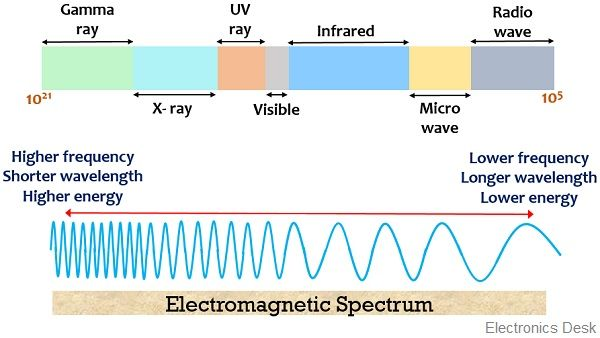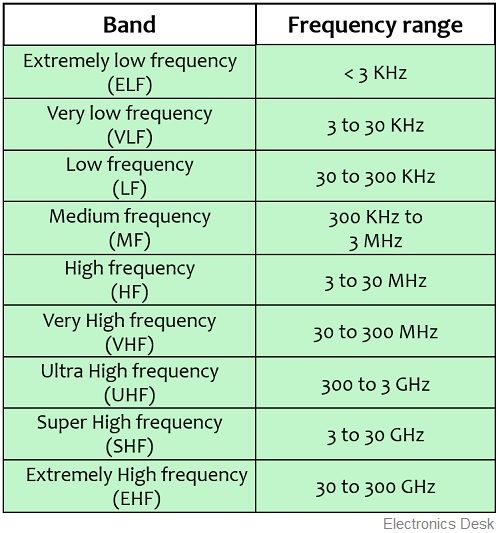Definition: A part of the electromagnetic spectrum that holds the least frequency range and highest wavelength is known as the radio frequency spectrum. It is sometimes referred as radio spectrum or radio wave spectrum. Generally, the radio spectrum has a frequency range between 30 kHz to 300 GHz.

We know that an electromagnetic spectrum contains a wide frequency range within it. And the radio frequency spectrum is a part of this electromagnetic spectrum. In the electromagnetic spectrum, energy travels in the form of waves.

So, we can say the radio wave is nothing but the waves that acquire the lowest-frequency portion in the electromagnetic spectrum.

## What is the radio spectrum?

We know that the electromagnetic spectrum contains a wide frequency range between 3 *105 to 3 * 1021 Hz.

We are already aware of the fact that frequency and wavelength are reciprocal of each other. Thus lower the frequency more will be the wavelength. Also, the product of frequency and wavelength provides the velocity of propagation of the wave. And we know that the propagation velocity in vacuum or air is around 3 * 108 m/s.

Therefore, the lower the frequency, the greater will be the wavelength and vice-versa.

So, radio waves occupy the lowest frequency range in the electromagnetic spectrum with greater wavelength. Hence it is said to be the lowest frequency region of the electromagnetic spectrum.

It has a wavelength range of 1 mm to 100 Km with frequency ranging between 30 kHz to 300 GHz.

Due to the extremely wide range of frequency of the electromagnetic spectrum, there are various other forms of electromagnetic waves, except the radio waves in the spectrum. These include infrared, visible, UV, microwave, x-rays, gamma rays, etc.

Despite having different names and being considered as a separate entity, these all are forms of electromagnetic energy. However, the fundamental difference between various types of waves is the different frequency range in which these waves lie.

Due to this they possess different characteristics and hence find applications in multiple fields.

Thus an electromagnetic spectrum is nothing but the representation of different electromagnetic waves according to their specific frequency and wavelength.

The figure below represents the various waves that are present in the electromagnetic spectrum:As we can see that electromagnetic spectrum holds a very large range of frequencies. However, even the radio waves have a quite large frequency range. Thus the entire frequency range of the radio wave spectrum is divided into different sections and each section is designated with a specific name.

The figure below shows the radio wave spectrum:Here as we can see that there exists a wide frequency range in the radio wave spectrum which is divided into various ranges.

As we have already discussed that the frequency range in the case of radio waves is 30 kHz to 300 GHz. So, in this frequency chart, 30 kHz frequency is mentioned in the low-frequency region of the spectrum. While 300 GHz is the extremely high-frequency region.  However, sometimes it is considered to be in the range from 30 Hz to 300 GHz i.e., considering even the very low-frequency range.

Basically these types of electromagnetic waves can be generated either naturally or artificially. The natural sources include lightning or astronomical phenomena. While artificial sources can be radar, satellites, radio towers, etc.

Radio waves are mainly classified as:

• AM radio waves: These are amplitude modulated radio waves in which the amplitude of the carrier wave is modulated according to the amplitude of the message signal. It carries communication radio signals in the range 540 to 1600 kHz.
• FM radio waves: These are frequency modulated radio waves where the frequency of the carrier wave is altered according to the modulating signal. However, FM radio waves have a frequency ranging from 88 to 108 MHz.

This is the reason why the FM radio broadcast systems have a frequency ranging between 88 to 108 MHz.

FM radio waves are less subjective to noise than AM radio waves. This is so because AM receivers interpret noise even as a part of the information signal. Since noise causes variation in amplitude thus FM is less prone to added noise because only frequency varies and amplitude remains constant in case of FM signals.

Suppose we have a radio station at 92.8 FM. So, this means that this radio station is broadcasting FM signals having a frequency of 92.8 MHz. This megahertz unit denotes the occurrence of millions of cycles in one second.

This simply shows that the transmitting antenna is sending a signal that is oscillating 92,800,000 cycles in each second. So, when the radio is tuned to that particular frequency, then the desired signal is received.

But if we talk about the broadcasting of AM radio wave signals then as the range is 535 kHz to 1600 kHz. So, the frequency of oscillation of the signal will be 535000 cycles in each second.

It is noteworthy here that radio waves of different frequencies possess distinct behavior while propagating in the atmosphere. Basically the waves having short frequency range propagates through ground wave propagation.

While comparatively higher frequency propagates, by getting reflected from the ionosphere, thereby allowing skywave propagation. However, even larger frequencies cover a greater portion of the earth and signal propagates through space wave propagation.

The penetration ability of radio waves depends on their wavelength. This means larger the wavelength, more will be the level of penetration and vice-versa.